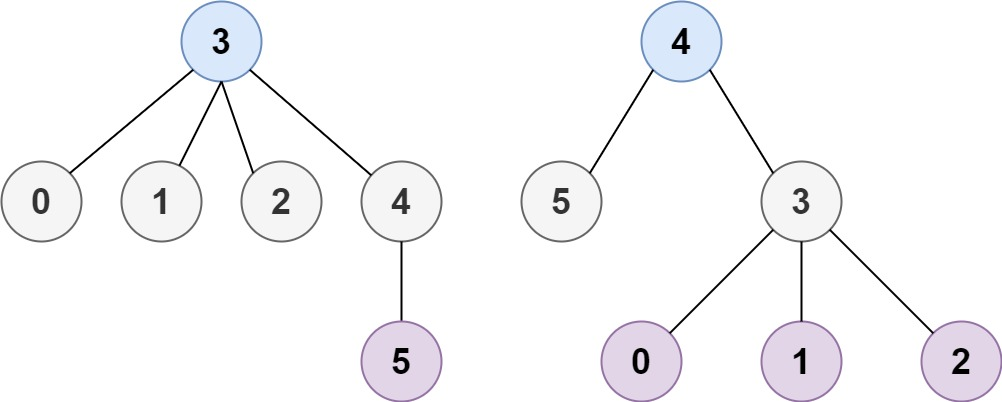304 North Cardinal St.
Dorchester Center, MA 02124

# Minimum Height Trees LeetCode Solution

## Problem – Minimum Height Trees LeetCode Solution

A tree is an undirected graph in which any two vertices are connected by exactly one path. In other words, any connected graph without simple cycles is a tree.

Given a tree of `n` nodes labelled from `0` to `n - 1`, and an array of `n - 1` `edges` where `edges[i] = [ai, bi]` indicates that there is an undirected edge between the two nodes `ai` and `bi` in the tree, you can choose any node of the tree as the root. When you select a node `x` as the root, the result tree has height `h`. Among all possible rooted trees, those with minimum height (i.e. `min(h)`)  are called minimum height trees (MHTs).

Return a list of all MHTs’ root labels. You can return the answer in any order.

The height of a rooted tree is the number of edges on the longest downward path between the root and a leaf.

Example 1:``````Input: n = 4, edges = [[1,0],[1,2],[1,3]]
Output: 
Explanation: As shown, the height of the tree is 1 when the root is the node with label 1 which is the only MHT.
``````

Example 2:``````Input: n = 6, edges = [[3,0],[3,1],[3,2],[3,4],[5,4]]
Output: [3,4]
``````

Constraints:

• `1 <= n <= 2 * 104`
• `edges.length == n - 1`
• `0 <= ai, bi < n`
• `ai != bi`
• All the pairs `(ai, bi)` are distinct.
• The given input is guaranteed to be a tree and there will be no repeated edges.

## Minimum Height Trees LeetCode Solution in Java

``````public List<Integer> findMinHeightTrees(int n, int[][] edges) {
if (n == 1) return Collections.singletonList(0);

for (int[] edge : edges) {
}

List<Integer> leaves = new ArrayList<>();
for (int i = 0; i < n; ++i)

while (n > 2) {
n -= leaves.size();
List<Integer> newLeaves = new ArrayList<>();
for (int i : leaves) {
}
leaves = newLeaves;
}
return leaves;
}

``````

## Minimum Height Trees LeetCode Solution in Python

``````def findMinHeightTrees(self, n, edges):
if n == 1: return 
adj = [set() for _ in xrange(n)]
for i, j in edges:

leaves = [i for i in xrange(n) if len(adj[i]) == 1]

while n > 2:
n -= len(leaves)
newLeaves = []
for i in leaves:
leaves = newLeaves
return leaves
``````

## Minimum Height Trees LeetCode Solution in C++

``````class Solution {
public:
vector<int> findMinHeightTrees(int n, vector<vector<int>>& edges) {
if(n==0)
return {};
if(n==1)
return {0};
vector<int>res;
vector<int>degrees(n,0);
for(int i=0;i<edges.size();i++)
{
degrees[edges[i]]++;//updating how many edges each node has
degrees[edges[i]]++;
}
queue<int>queue;
for(int i=0;i<n;i++)
{
queue.push(i);
}
while(!queue.empty())
{
res.clear();// clear vector before we start traversing level by level.
int size=queue.size();
for(int i=0;i<size;i++)
{
int cur=queue.front();
queue.pop();
res.push_back(cur);//adding nodes to vector.Goal is to get a vector of  just 1 or 2 nodes available.
{
degrees[neighbor]--;//removing current leave nodes
queue.push(neighbor);
}
}
}
return res;
}
};
``````
##### Minimum Height Trees LeetCode Solution Review:

In our experience, we suggest you solve this Minimum Height Trees LeetCode Solution and gain some new skills from Professionals completely free and we assure you will be worth it.

If you are stuck anywhere between any coding problem, just visit Queslers to get the Minimum Height Trees LeetCode Solution

Find on Leetcode

##### Conclusion:

I hope this Minimum Height Trees LeetCode Solution would be useful for you to learn something new from this problem. If it helped you then don’t forget to bookmark our site for more Coding Solutions.

This Problem is intended for audiences of all experiences who are interested in learning about Data Science in a business context; there are no prerequisites.

Keep Learning!

More Coding Solutions >>

LeetCode Solutions

Hacker Rank Solutions

CodeChef Solutions